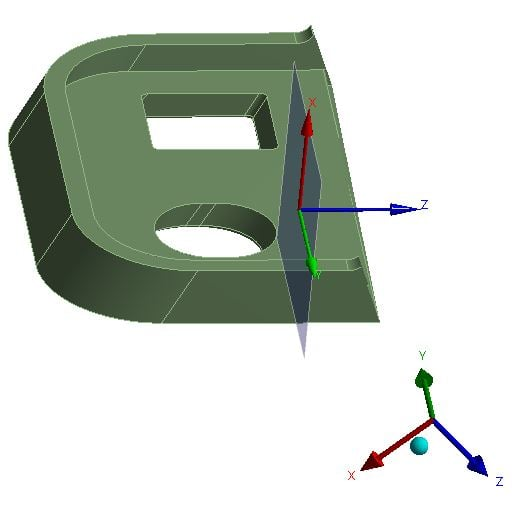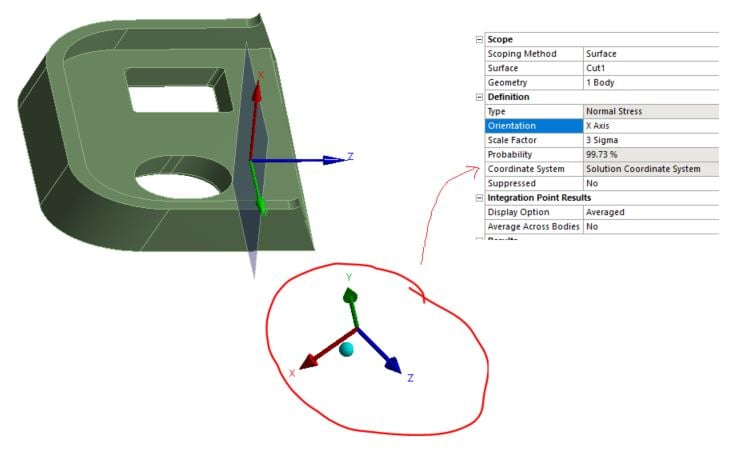## General Mechanical

•Autonewbie
Subscriber

Hi,

I would like to ask how to calculate membrane stress under Random Vib (MSUP)? I have defined the surface but it seems missing something... Thanks!

•Autonewbie
Subscriber

https://www.simutechgroup.com/tips-and-tricks/fea-articles/118-fea-tips-tricks-stress-linearization

With refer to this article, it seems that I have to use APDL command to perform surface operation for nominal stress.

•peteroznewman
Subscriber

Please show what your geometry looks like.  Is it a surface covered in shell elements?  Did you know that the 11 direction of elements can be quite random on a surface?  You can correct that by assigning an element coordinate system.

•Autonewbie
Subscriber

Hi Peter,

I figure it out. I was using surface operation in APDL but I did not know that membrane stress option is for shell element only (I assume) as no warning message given. So, I create a surface at the location of interest in solid model and extract the normal stress then take the average of the max/min stress. That is the nominal stress/ membrane stress. I crossed check with hand calculation and surface operation in APDL also.

•Autonewbie
Subscriber

Hi Peter,

I would like to extract the normal stress to the surface created but none of the solution coordination axis is normal to the surface. How do we can the normal stress in the desired direction in random vibration? Thank you!•peteroznewman
Subscriber

When you request a Normal Stress, you get to choose the coordinate system you want to use. Just select the one that is aligned normal to that plane.

•Autonewbie
Subscriber

In Random Vib, I cannot choose the coordinate system...and the cutting plan is not aligned to the global coordinate system which is used in solution.•peteroznewman
Subscriber

Sorry, I didn't have a Random Vibration model to open to check my answer, which works in Static Structural models.  I will have to make a Random Vibration model, or you can attach one for me to look at.

•peteroznewman
Subscriber

I pulled up the Dynamics training course and reviewed the Random Vibration class.  Below is a relevant slide.

The important point is that the directional results are statistical in nature and cannot be combined in the usual way.
This has something to do with the fact that Normal Stress is only available in the Solution Coordinate System.

•Autonewbie
Subscriber

Hi, do you still need a model from me? I can prepare a modified model if you are still interested.

Besides, thank you for taking up time to check the training materials.

Is it possible to change the solution coordinate system upfront during modal analysis? But it seems not efficient as we will only know where the cross section to be made after knowing the critical area from the result.

•peteroznewman
Subscriber

I made my own simple model with Random Vibration, so I am all set.

A workaround to get Normal Stress in a particular direction is to go back to CAD and rotate the geometry to get the plane aligned with a global axis.  I don't know if there is a way to change the Solution Coordinate System.

•Autonewbie
Subscriber

Thanks Peter!

I am trying to see if it is feasible to transform the stress to the direction by rotating the model but with a new simple model.

It would be better if we can transform the stress by doing math as rotate the whole CAD and re-run the Modal and RV is not an ideal way, the modal usually take few hours to run ...

•peteroznewman
Subscriber

Even if you rotate the geometry, there is another potential issue, the PSD loads can only be applied along the global axes.

If you had a model on a single axis shaker table, and the assembly sat on the table such that the original global Y axis was the one getting a PSD acceleration profile, after you rotate the model by 45 degrees for example, you can't apply the PSD acceleration in the same direction as it was before.

Maybe you need to reconsider the way you evaluate the stress in the part. What if you use Equivalent Stress instead of Normal Stress?

•Autonewbie
Subscriber

I observed the same issue as you said. I was making a model and apply the PSD load which is at the third direction (Z-direction, rotate in X direction), then trying to convert the stress to 45 degree and verify it again...

I am not sure if equivalent stress is appropriate as I want to find out the normal stress to avoid peak stress that is excluded in the assessment.

•peteroznewman
Subscriber

I don't understand exactly what you mean by this, "I want to find out the normal stress to avoid peak stress that is excluded in the assessment."

If you want to explain that further, I am interested to understand what you are trying to do with this simulation.

•Autonewbie
Subscriber

Currently, the max stress observed in Random Vibration exceeds yield. However, the maximum stress is the peak stress and it is usually will be re-distributed at plastic region. So, we will assess it by looking at the nominal stress which is excluding peak stress in the solid modeling (equivalent to membrane stress in shell modeling).

However, this method is common in static analysis but I am not sure if it is suitable for dynamics analysis ( I am new to dynamics), particularly for random vibration. Correct me if the assumption is not realistic. Thanks.

•peteroznewman
Subscriber

Random Vibration (RV) is a linear model, so the material model that is used during the RV solution is only linear elastic, and no plasticity is used even if it is in the material definition. You understand that any region that exceeds yield stress will release stress by plastic deformation in the real part, though that will not be represented in the RV simulation.

One way to evaluate the effect of a small region of material exceeding yield is to select those elements and assign them a different material that has a lower Young's Modulus, then rerun the analysis. This will result in lower stress in that region while simultaneously transferring more load to the surrounding material.

You should understand that the results from a RV analysis are statistical quantities. The results are 1 sigma or 3 sigma levels, therefore, you should not use the word "peak" when referring to RV results.  There is a maximum 3-sigma stress in the model.  That means you expect the values of stress at the location of the maximum to be less than the 3-sigma value 99.865% of the time, but a small percentage of the time, 0.135%, the stress could exceed the 3-sigma value.

•Autonewbie
Subscriber

Yes. We do not use plastic material properties into Random Vibration Analysis. What I am saying is the 'peak' stress will eventually re-distributed and therefore we look at nominal stress. However, I am concerned of RV analysis is statistical quantities. So, I am figuring what assumptions are more realistic to use.

I read up a manual that saying two general methods used to assess dynamics analysis: convert the dynamics load to equivalent static load and another is fatigue. Does it mean using the max stress from Random Vibration Analysis against with material strength is not realistic except performing RV Fatigue? Thanks!

•peteroznewman
Subscriber

How much past yield did the stress go?  20% is probably okay, 200% might be a concern.

There are two types of failure: Overload and Fatigue.

Overload means the part breaks after a single application of load. That overload might happen at the peak load of a dynamic event like an earthquake, or the impact of the product with the floor when it is dropped. The evaluation of the peak stress can help to decide if failure is likely to occur due to overload by comparison with the ultimate strength for brittle materials or by comparison of the total strain with the elongation at break for ductile materials.

Fatigue means the part breaks after many thousands or even millions of cycles of load, where the peak stress is usually below the yield strength. The stress amplitude and mean stress are compared with the material SN curve to decide how many cycles are needed to get to a 50% probability of failure.  Random vibration testing is often used to generate thousands or millions of cycles of load in a few hours. The amplitude of the stress cycle depends on the modal frequencies of the structure, the damping of the structure and the input PSD as to whether a resonant frequency is being excited by the PSD input load.

•Autonewbie
Subscriber

The safety factor is about ~2.5 with yield strength... But it is localized or...that is the reason I was thinking to check the nominal stress or thinking to use Neuber rule... but it does very high ratio to me to think of doing random vibration fatigue instead...

•peteroznewman
Subscriber

I'm confused.  Safety Factor = Yield_Strength/Stress.  So a Safety Factor of 2.5 is way below Yield.  Do you mean the inverse of Safety Factor?  That the stress is 2.5 times yield?

Please insert an image of where the stress is above yield.  Scale the legend so that the transition value from orange to red is set to the yield strength. That way, any material above yield is colored red.  You can have a second image that is a Section view to show how deep into the material the yielding goes.

•Autonewbie
Subscriber

Sorry Peter. I calculated it wrongly... SF is about 0.38.

That is the reason I want to predict the nominal stress to see how much of the stress that above yield at the cross section. I prefer to have the number instead of just assessing the stress contour (stress> YS) as it is very marginal or could possibly fail.

However, at least, I understand that it might not be feasible to do so under statistic analysis.

•peteroznewman
Subscriber

One way to evaluate the effect of a small region of material exceeding yield in a linear analysis like Random Vibration is to select those elements and assign them a different material that has a lower Young's Modulus, then rerun the analysis. This will result in lower stress in that region while simultaneously transferring more load to the surrounding material.  You might need some Named Selections and a Command object to change the material properties on a few elements.

•peteroznewman
Subscriber

Instead of that, you can slice the solid body and use Shared Topology to keep it connected, but now you can assign a softer material to the region that you know exceeded yield strength to assess the structural integrity of that part of the model after it has lost some load carrying capacity due to plasticity.